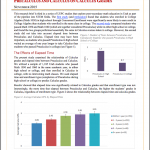## Precalculus to Calculus DelayThis study used a sample of 1,185 Utah students to examine the relationship of elapsed time between Precalculus and Calculus on Calculus grades.

• Results indicated that waiting longer to take Calculus after Precalculus was related to higher Calculus grades.
• This finding could not be explained by the relationship of elapsed time to any of the other student variables.
Precalculus to Calculus Delay Full Study